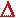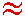Math & Science Home | Proficiency Tests | Mathematical Thinking in Physics | Aeronauts 2000

 CONTENTS Introduction Fermi's Piano Tuner Problem How Old is Old? If the Terrestrial Poles were to Melt... Sunlight Exerts Pressure Falling Eastward What if an Asteroid Hit the Earth Using a Jeep to Estimate the Energy in Gasoline How do Police Radars really work? How "Fast" is the Speed of Light? How Long is a Light Year? How Big is a Trillion? "Seeing" the Earth, Moon, and Sun to Scale Of Stars and Drops of Water If I Were to Build a Model of the Cosmos... A Number Trick Designing a High Altitude Balloon Pressure in the Vicinity of a Lunar Astronaut Space Suit due to Outgassing of Coolant Water Calendar Calculations Telling Time by the Stars - Sidereal Time Fields, an Heuristic Approach The Irrationality ofThe Irrationality ofThe Number (i)i Estimating the Temperature of a Flat Plate in Low Earth Orbit Proving that (p)1/n is Irrational when p is a Prime and n>1 The Transcendentality ofIdeal Gases under Constant Volume, Constant Pressure, Constant Temperature and Adiabatic Conditions Maxwell's Equations: The Vector and Scalar Potentials A Possible Scalar Term Describing Energy Density in the Gravitational Field A Proposed Relativistic, Thermodynamic Four-Vector Motivational Argument for the Expression-eix=cosx+isinx Another Motivational Argument for the Expression-eix=cosx+isinx Calculating the Energy from Sunlight over a 12 hour period Calculating the Energy from Sunlight over actual full day Perfect Numbers-A Case Study Gravitation Inside a Uniform Hollow Sphere Further note on Gravitation Inside a Uniform Hollow Sphere Pythagorean Triples Black Holes and Point Set Topology Additional Notes on Black Holes and Point Set Topology Field Equations and Equations of Motion (General Relativity) The observer in modern physics A Note on the Centrifugal and Coriolis Accelerations as Pseudo Accelerations - PDF File On Expansion of the Universe - PDF File

## If the Terrestrial Poles Were to Melt...

Problem:
If the terrestrial poles were to melt, then estimate how much the earth's oceans would rise.

Solution:
If the poles melted, then

1. The north polar ice would make essentially no contribution since it is float ice.
2. The south polar ice would make a considerable contribution since it overlays the Antarctic land mass and is not float ice.

First, let us estimate the total volume of the south polar ice. We begin by noting that, on the Earth, 1 degree of latitude corresponds to a distance of 69.2 mi. Now, using a well marked map, Antarctica may be estimated as having an angular radius of 18 degrees of latitude, or a radius in miles of rA = 69.2 mi/deg x 18 deg = 1.25 x 103 mi. Its estimated surface area is thenrA2 = 4.91 x 106 mi2. If we assume the average thickness of the Antarctic ice to be 1 mi, then the total ice volume is

VIce = 4.91 x 106 mi3.

If this ice melts, the resulting volume of liquid water is 4/5 of this value or

VWater = 3.93 x 106 mi3.

Now, let this volume of water be spread in a uniform, thin shell over a uniform Earth (for the moment, we will ignore the continents). The total surface area of the Earth is AE = 4rE2 = 1.97 x 108 mi2, where rE = 3.96 x 103 mi. Since the thickness of this shell,rE, may be assumed to be << the Earth's radius, rE , we may write

3.93 x 106 mi3AErE1

or,rE = 1.99 x 10-2 mi = 105 ft.

If we now return the continents to their proper place, it is easy to see that the value ofrE must become larger. Since only 2/3 of the earth's surface area is ocean, the thickness of the water shell must be increased by a factor of about 3/2:rE = (3/2) x 105 ft = 158 ft. 2

Thus, we have estimated that if the polar ice were to melt, the Earth's oceans would rise by about 150 ft. Reference to a map showing land elevations allows us to determine that coastal areas and major river valleys (such as the Mississippi or the Amazon river valleys) would be flooded. The land distribution would appear somewhat differently than it does on the globe today. But the majority of land would still be above sea level.

1
Actually the volume of the water shell is VWater = (4/3)(r23 - r13), where r1 is the radius of the Earth prior to flooding (3.96 x 103 mi), and r2 is the radius of the Earth after flooding (r2 = r1 +rE). We may simplify the above expression for VWater to read VWater = (4/3)(rE3), where(rE3) = (r1 +rE)3 - r13= 3r2rE + 3r1(rE)2 + (rE)33r2rE, sincerE << r1. Thus VWater4r2rE = AErE. This last expression is the one given in the text.

2 The actual volume of Antarctic ice is 7 x 106 mi3. Using this value rather than the value 4.91 x 106 mi3 previously calculated, we findrE = 225 ft., close to the values given in text books.